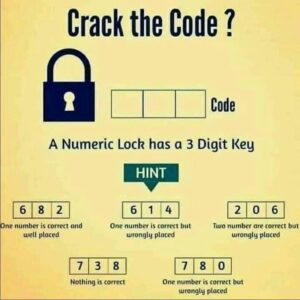# ExplanationExplanation

We get the first idea about the first number from hint 4. It makes clear that 7, 3 & 8 are not a part of the key.

Now, look at the 5th hint. It says ‘one number is correct but wrongly placed.’ From hint 4 we know that 7 & 8 are not involved in the key. So, the number is 0 but it is wrongly placed.

Now look at hint 1. It says ‘one number is correct and well placed.’ We know that 8 is not that number. So it can either be 6 or 2.

Now look at hint no. 3. It says ‘two numbers are correct but wrongly placed’. Now we know one of those two numbers is 0. So, if it is wrongly placed in hint 3 and also wrongly placed in hint 5 then it means that it should be placed at the first position. Hence the first digit of the code is 0.

Also, we come to know that the next correct number is 2 and not 6. Because, if we see hint 1 and 3, we realize that 0 is the first digit of the code. Hence, 6 cannot be the first digit. So, from the first hint, 2 is the third digit.

Now remains the middle digit. If you look at the 2nd hint it says ‘one number is correct but wrongly placed’ which means the middle digit is 4. We already know 6 is not involved in the key. Therefore, 4 is the only digit which is wrongly placed in that key.

Hence, the key is 042.